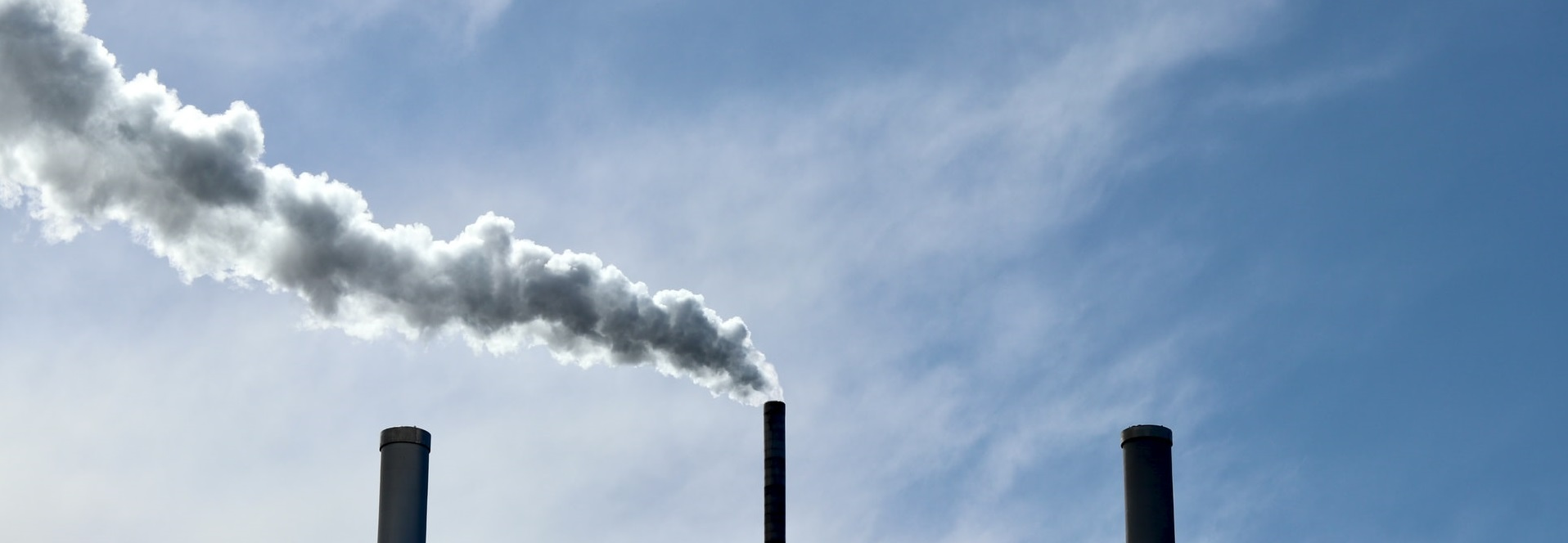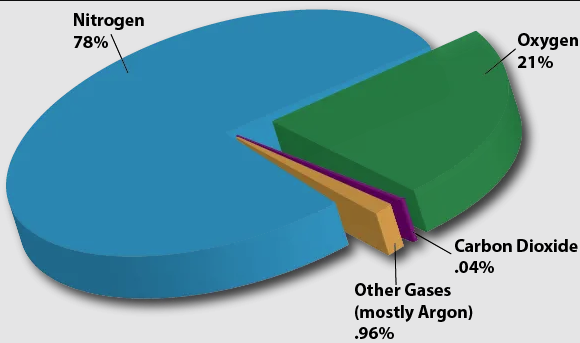# Stack Gas Volumetric Flow Correction CalculatorPhoto by Finn Mund on Unsplash

## Correction of Volumetric Flow

In the measurement of stack gas volumetric flow rates and pollutant concentrations, there are four crucial considerations:

• Temperature;
• Pressure;
• Moisture content;
• Excess oxygen.

Each of these parameters influence the volume of the stack exhaust gas as described by either Boyles law or Avogadro’s law. Therefore, different conditions would yield different volumetric flows – a critical consideration in stack gas pollutant concentration tests. See our stack gas concentration measurement correction calculator to adjust or correct measured concentrations based on temperature, pressure, moisture and oxygen content.

Use the stack gas volumetric flow correction calculator below to correct measured volumetric flow from measurement conditions to reference conditions.

## How do temperature and pressure influence gas volume?

From Boyles ideal gas law we know that at a constant temperature, the pressure of a given amount of gas is inversely proportional to its volume. By manipulating the ideal gas law formula it can be shown that the volume of a gas is directly proportional to temperature, and inversely proportional to pressure:

pv = k = nRT

v = nRT/p

v ∝ T

v1/p

Therefore, in the context of stack gas measurements and testing, factoring actual conditions in the measurement of volumetric flow and subsequent calculations of the pollutant concentrations is vital. For example, as the temperature of a gas increases, its volume increases – and the higher volumetric flow rate would mean a concentration measurement would be lower. Inversely, at a lower altitude where atmospheric pressure is relatively higher, lower volumetric flow rates would be measured resulting in higher measured concentrations.

## How does moisture content affect gas volume?

Moisture or water (H₂O) is a common product in fossil fuel combustion, and can occupy a significant fraction of the exhaust gas volume. Therefore, the higher the moisture content, the higher the volumetric flow. Usually emission limits and emission measurements are expressed on a dry basis, and “wet” measurements need to be corrected to “dry” volumetric flow.

To correct the measured “wet” volume to a “dry” volume, the “wet” volume is factored by the difference between total and H₂O volume percentage as follows:

Dry Volume = Wet Volume × (100 – %H₂O)/(100)

## How does excess oxygen affect volume?

The effect of excess oxygen on measured exhaust gas volume is somewhat more subtle. The key difference is that excess oxygen means that there is excess air flow, and excess air flow means excess oxygen, nitrogen and other trace gasses. Air consists of approximately 21% oxygen, 78% nitrogen and the remaining 1% is occupied by trace gasses.Credit: UCAR

Therefore, the formula for oxygen correction accounts for the additional 78% nitrogen and 1% trace gasses. With a bit of mathematical manipulation, the formula for oxygen correction can be derived and simplified, resulting in the following formula:

vat ref. conditions = vat measured conditions × (20.9 – measured excess O₂)/(20.9 – ref. excess O₂)